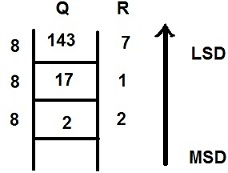## Decimal - Octal Converter

 Enter Decimal Number Select Conversion Decimal to Octal Octal to Decimal
 Result: Octal

The Decimal - Octal Converter could convert Decimal to Octal or Octal to Decimal. It is very easy to use.

For example, when you enter a decimal number is 928, then the equivalent octal number is 1640.

When you enter a octal number is 77, then the equivalent decimal number is 63.

The Fig below shows how to convert decimal number 143 into its equivalent octal number:If you want to convert Octal number 143 to its decimal

Steps = 1 x 82 + 4 x 81 + 3 x 80
= 1 x 64 + 4 x 8 + 3 x 1
= 64 + 32 + 3
= 99

So the equivalent decimal number is 99

Thinkcalculator.com provides you helpful and handy calculator resources.